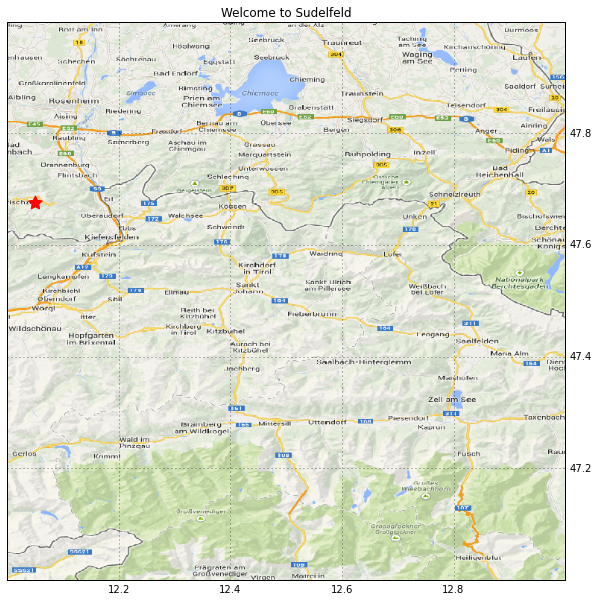# Shaded Relief Map in Python¶

Author: Thomas Lecocq

Some standard and some less-standard imports:

In :
import matplotlib.pyplot as plt
import numpy as np

import cartopy.crs as ccrs
from cartopy.io.srtm import srtm_composite

from osgeo import gdal
from osgeo import gdal_array


Reasons for each packages:

• cartopy: base of all our maps, handles the projections and transforms + provides automatic download features for many cool stuff (Google Maps tiles, SRTM data, etc)
• osgeo: needed to clean buggy SRTM data

## First Map¶

I am currently in Sudelfeld for a workshop organised by the colleagues of Munich University. Let's plot a 1-degree square image comprising the village we are in. The Google Maps tiles are downloaded automatically from the webservice (so an Internet connection is needed):

In :
# Specify a region of interest, in this case, Sudelfeld Ski Resort (Germany)
lat = 47 + 40 / 60.0 + 30 / 3600.
lon = 12 + 3 / 60.0 + 2 / 3600.

plt.figure(figsize=(10, 10))
ax = plt.subplot(111, projection=ccrs.PlateCarree())
ax.set_extent([12.0, 13.0, 47.0, 48.0])

plt.scatter(lon, lat, marker=(5, 1), color='red', s=200)
plt.title("Welcome to Sudelfeld")
gl = ax.gridlines(draw_labels=True,)
gl.xlabels_top = False
gl.ylabels_left = False## Second Map: SRTM elevation¶

SRTM data can be fetched automatically like the tiles, and plotted using imshow:

In :
plt.figure(figsize=(15, 10))
ax = plt.subplot(111, projection=ccrs.PlateCarree())

elev, crs, extent = srtm_composite(12, 47, 1, 1)
plt.imshow(elev, extent=extent, transform=crs,
cmap='gist_earth', origin='lower')
cb = plt.colorbar(orientation='vertical')
cb.set_label('Altitude')
plt.title("SRTM Map")
gl = ax.gridlines(draw_labels=True,)
gl.xlabels_top = False
gl.ylabels_left = False